## Faster R-CNN Examples

### Create R-CNN Object Detection Network

This example shows how to modify a pretrained ResNet-50 network into an R-CNN object detection network. The network created in this example can be trained using `trainRCNNObjectDetector`.

```% Load pretrained ResNet-50. net = resnet50(); % Convert network into a layer graph object to manipulate the layers. lgraph = layerGraph(net);```

The procedure to convert a network into an R-CNN network is the same as the transfer learning workflow for image classification. You replace the last 3 classification layers with new layers that can support the number of object classes you want to detect, plus a background class.

In ResNet-50, the last three layers are named fc1000, fc1000_softmax, and ClassificationLayer_fc1000. Display the network, and zoom in on the section of the network you will modify.

```figure plot(lgraph) ylim([-5 16])```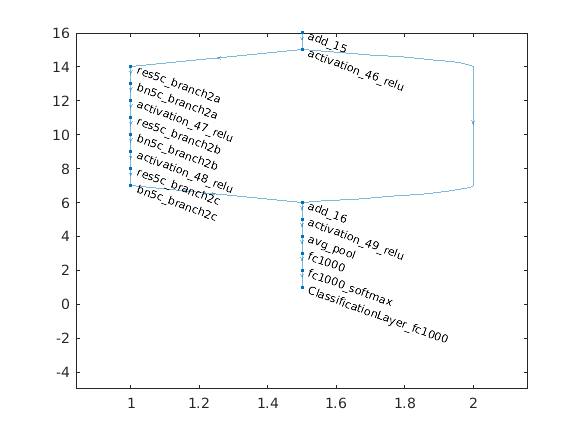```% Remove the last 3 layers. layersToRemove = { 'fc1000' 'fc1000_softmax' 'ClassificationLayer_fc1000' }; lgraph = removeLayers(lgraph, layersToRemove); % Display the results after removing the layers. figure plot(lgraph) ylim([-5 16])```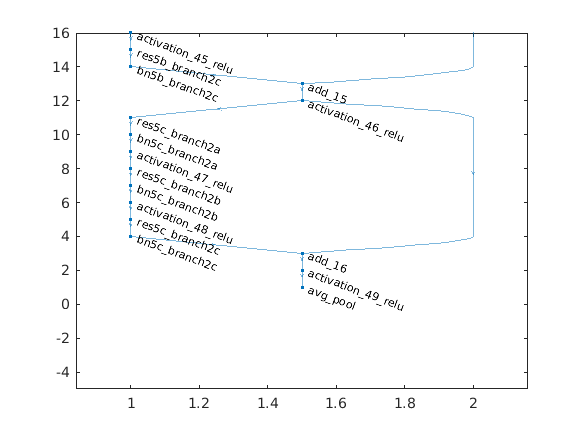Add the new classification layers to the network. The layers are setup to classify the number of objects the network should detect plus an additional background class. During detection, the network processes cropped image regions and classifies them as belonging to one of the object classes or background.

```% Specify the number of classes the network should classify. numClassesPlusBackground = 2 + 1; % Define new classfication layers newLayers = [ fullyConnectedLayer(numClassesPlusBackground, 'Name', 'rcnnFC') softmaxLayer('Name', 'rcnnSoftmax') classificationLayer('Name', 'rcnnClassification') ]; % Add new layers lgraph = addLayers(lgraph, newLayers); % Connect the new layers to the network. lgraph = connectLayers(lgraph, 'avg_pool', 'rcnnFC'); % Display the final R-CNN network. This can be trained using trainRCNNObjectDetector. figure plot(lgraph) ylim([-5 16])```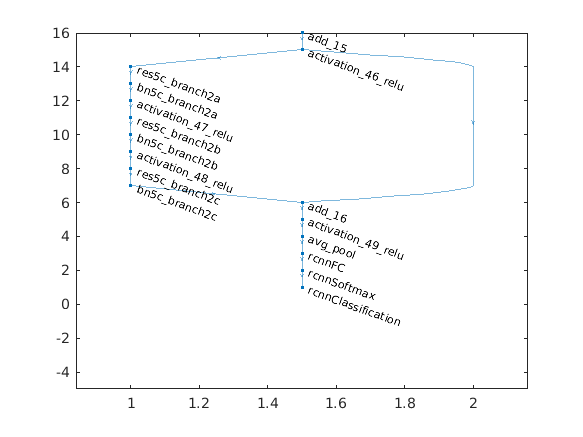### Create Fast R-CNN Object Detection Network

This example builds upon the Create R-CNN Object Detection Network example above. It transforms a pretrained ResNet-50 network into a Fast R-CNN object detection network by adding an ROI pooling layer and a bounding box regression layer. The Fast R-CNN network can then be trained using `trainFastRCNNObjectDetector`.

Create R-CNN Network

Start by creating an R-CNN network that forms the basis of Fast R-CNN. The Create R-CNN Object Detection Network example explains this section of code in detail.

```% Load pretrained ResNet-50. net = resnet50; lgraph = layerGraph(net); % Remove the the last 3 layers from ResNet-50. layersToRemove = { 'fc1000' 'fc1000_softmax' 'ClassificationLayer_fc1000' }; lgraph = removeLayers(lgraph, layersToRemove); % Specify the number of classes the network should classify. numClasses = 2; numClassesPlusBackground = numClasses + 1; % Define new classification layers. newLayers = [ fullyConnectedLayer(numClassesPlusBackground, 'Name', 'rcnnFC') softmaxLayer('Name', 'rcnnSoftmax') classificationLayer('Name', 'rcnnClassification') ]; % Add new layers. lgraph = addLayers(lgraph, newLayers); % Connect the new layers to the network. lgraph = connectLayers(lgraph, 'avg_pool', 'rcnnFC');```

Add a box regression layer to learn a set of box offsets to apply to the region proposal boxes. The learned offsets transform the region proposal boxes so that they are closer to the original ground truth bounding box. This transformation helps improve the localization performance of Fast R-CNN.

The box regression layers are composed of a fully connected layer followed by an R-CNN box regression layer. The fully connected layer is configured to output a set of 4 box offsets for each class. The background class is excluded because the background bounding boxes are not refined.

```% Define the number of outputs of the fully connected layer. numOutputs = 4 * numClasses; % Create the box regression layers. boxRegressionLayers = [ fullyConnectedLayer(numOutputs,'Name','rcnnBoxFC') rcnnBoxRegressionLayer('Name','rcnnBoxDeltas') ]; % Add the layers to the network lgraph = addLayers(lgraph, boxRegressionLayers);```

The box regression layers are typically connected to same layer the classification branch is connected to.

```% Connect the regression layers to the layer named 'avg_pool'. lgraph = connectLayers(lgraph,'avg_pool','rcnnBoxFC'); % Display the classification and regression branches of Fast R-CNN. figure plot(lgraph) ylim([-5 16])```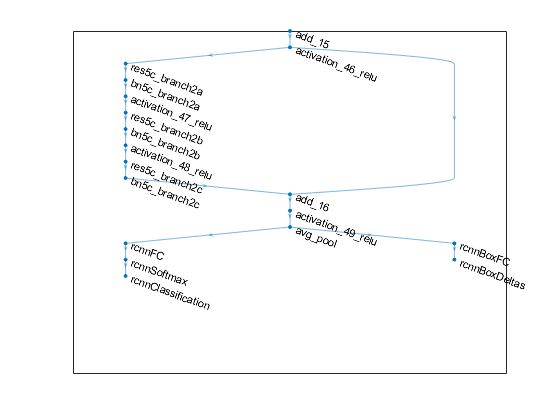The next step is to choose which layer in the network to use as the feature extraction layer. This layer will be connected to the ROI max pooling layer which will pool features for classifying the pooled regions. Selecting a feature extraction layer requires empirical evaluation. For ResNet-50, a typical feature extraction layer is the output of the 4-th block of convolutions, which corresponds to the layer named activation40_relu.

```featureExtractionLayer = 'activation_40_relu'; figure plot(lgraph) ylim([30 42])```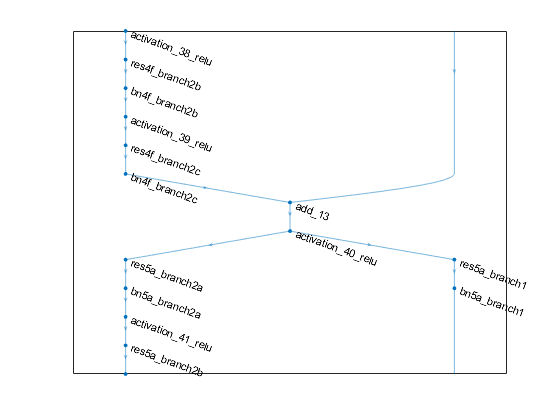In order to insert the ROI max pooling layer, first disconnect the layers attached to the feature extraction layer: res5a_branch2a and res5a_branch1.

```% Disconnect the layers attached to the selected feature extraction layer. lgraph = disconnectLayers(lgraph, featureExtractionLayer,'res5a_branch2a'); lgraph = disconnectLayers(lgraph, featureExtractionLayer,'res5a_branch1'); % Add ROI max pooling layer. outputSize = [14 14]```
```outputSize = 1×2 14 14 ```
```roiPool = roiMaxPooling2dLayer(outputSize,'Name','roiPool'); lgraph = addLayers(lgraph, roiPool); % Connect feature extraction layer to ROI max pooling layer. lgraph = connectLayers(lgraph, 'activation_40_relu','roiPool/in'); % Connect the output of ROI max pool to the disconnected layers from above. lgraph = connectLayers(lgraph, 'roiPool','res5a_branch2a'); lgraph = connectLayers(lgraph, 'roiPool','res5a_branch1'); % Show the result after adding and connecting the ROI max pooling layer. figure plot(lgraph) ylim([30 42])```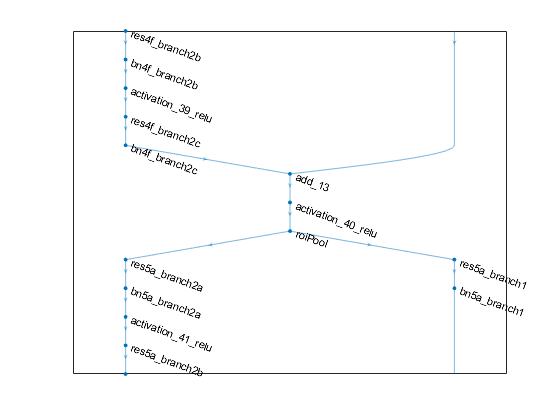Finally, connect the ROI input layer to the second input of the ROI max pooling layer.

```% Add ROI input layer. roiInput = roiInputLayer('Name','roiInput'); lgraph = addLayers(lgraph, roiInput); % Connect ROI input layer to the 'roi' input of the ROI max pooling layer. lgraph = connectLayers(lgraph, 'roiInput','roiPool/roi'); % Show the resulting faster adding and connecting the ROI input layer. figure plot(lgraph) ylim([30 42])```The network is ready to be trained using `trainFastRCNNObjectDetector`.

### Create Faster R-CNN Object Detection Network

This example builds upon the Create Fast R-CNN Object Detection Network example above. It transforms a pretrained ResNet-50 network into a Faster R-CNN object detection network by adding an ROI pooling layer, a bounding box regression layer, and a region proposal network (RPN). The Faster R-CNN network can then be trained using `trainFasterRCNNObjectDetector`.

Create Fast R-CNN Network

Start by creating Fast R-CNN, which forms the basis of Faster R-CNN. The Create Fast R-CNN Object Detection Network example explains this section of code in detail.

```% Load a pretrained ResNet-50. net = resnet50; lgraph = layerGraph(net); % Remove the last 3 layers. layersToRemove = { 'fc1000' 'fc1000_softmax' 'ClassificationLayer_fc1000' }; lgraph = removeLayers(lgraph, layersToRemove); % Specify the number of classes the network should classify. numClasses = 2; numClassesPlusBackground = numClasses + 1; % Define new classification layers. newLayers = [ fullyConnectedLayer(numClassesPlusBackground, 'Name', 'rcnnFC') softmaxLayer('Name', 'rcnnSoftmax') classificationLayer('Name', 'rcnnClassification') ]; % Add new object classification layers. lgraph = addLayers(lgraph, newLayers); % Connect the new layers to the network. lgraph = connectLayers(lgraph, 'avg_pool', 'rcnnFC'); % Define the number of outputs of the fully connected layer. numOutputs = 4 * numClasses; % Create the box regression layers. boxRegressionLayers = [ fullyConnectedLayer(numOutputs,'Name','rcnnBoxFC') rcnnBoxRegressionLayer('Name','rcnnBoxDeltas') ]; % Add the layers to the network. lgraph = addLayers(lgraph, boxRegressionLayers); % Connect the regression layers to the layer named 'avg_pool'. lgraph = connectLayers(lgraph,'avg_pool','rcnnBoxFC'); % Select a feature extraction layer. featureExtractionLayer = 'activation_40_relu'; % Disconnect the layers attached to the selected feature extraction layer. lgraph = disconnectLayers(lgraph, featureExtractionLayer,'res5a_branch2a'); lgraph = disconnectLayers(lgraph, featureExtractionLayer,'res5a_branch1'); % Add ROI max pooling layer. outputSize = [14 14]; roiPool = roiMaxPooling2dLayer(outputSize,'Name','roiPool'); lgraph = addLayers(lgraph, roiPool); % Connect feature extraction layer to ROI max pooling layer. lgraph = connectLayers(lgraph, featureExtractionLayer,'roiPool/in'); % Connect the output of ROI max pool to the disconnected layers from above. lgraph = connectLayers(lgraph, 'roiPool','res5a_branch2a'); lgraph = connectLayers(lgraph, 'roiPool','res5a_branch1'); ```

Faster R-CNN uses a region proposal network (RPN) to generate region proposals. An RPN produces region proposals by predicting the class, “object” or “background”, and box offsets for a set of predefined bounding box templates known as "anchor boxes". Anchor boxes are specified by providing their size, which is typically determined based on a priori knowledge of the scale and aspect ratio of objects in the training dataset.

Define the anchor boxes and create a `regionProposalLayer`.

```% Define anchor boxes. anchorBoxes = [ 16 16 32 16 16 32 ]; % Create the region proposal layer. proposalLayer = regionProposalLayer(anchorBoxes,'Name','regionProposal'); lgraph = addLayers(lgraph, proposalLayer);```

Add the convolution layers for RPN and connect it to the feature extraction layer selected above.

```% Number of anchor boxes. numAnchors = size(anchorBoxes,1); % Number of feature maps in coming out of the feature extraction layer. numFilters = 1024; rpnLayers = [ convolution2dLayer(3, numFilters,'padding',[1 1],'Name','rpnConv3x3') reluLayer('Name','rpnRelu') ]; lgraph = addLayers(lgraph, rpnLayers); % Connect to RPN to feature extraction layer. lgraph = connectLayers(lgraph, featureExtractionLayer, 'rpnConv3x3');```

Add the RPN classification output layers. The classification layer classifies each anchor as "object" or "background".

```% Add RPN classification layers. rpnClsLayers = [ convolution2dLayer(1, numAnchors*2,'Name', 'rpnConv1x1ClsScores') rpnSoftmaxLayer('Name', 'rpnSoftmax') rpnClassificationLayer('Name','rpnClassification') ]; lgraph = addLayers(lgraph, rpnClsLayers); % Connect the classification layers to the RPN network. lgraph = connectLayers(lgraph, 'rpnRelu', 'rpnConv1x1ClsScores');```

Add the RPN regression output layers. The regression layer predicts 4 box offsets for each anchor box.

```% Add RPN regression layers. rpnRegLayers = [ convolution2dLayer(1, numAnchors*4, 'Name', 'rpnConv1x1BoxDeltas') rcnnBoxRegressionLayer('Name', 'rpnBoxDeltas'); ]; lgraph = addLayers(lgraph, rpnRegLayers); % Connect the regression layers to the RPN network. lgraph = connectLayers(lgraph, 'rpnRelu', 'rpnConv1x1BoxDeltas'); ```

Finally, connect the classification and regression feature maps to the region proposal layer inputs, and the ROI pooling layer to the region proposal layer output.

```% Connect region proposal network. lgraph = connectLayers(lgraph, 'rpnConv1x1ClsScores', 'regionProposal/scores'); lgraph = connectLayers(lgraph, 'rpnConv1x1BoxDeltas', 'regionProposal/boxDeltas'); % Connect region proposal layer to roi pooling. lgraph = connectLayers(lgraph, 'regionProposal', 'roiPool/roi'); % Show the network after adding the RPN layers. figure plot(lgraph) ylim([30 42])```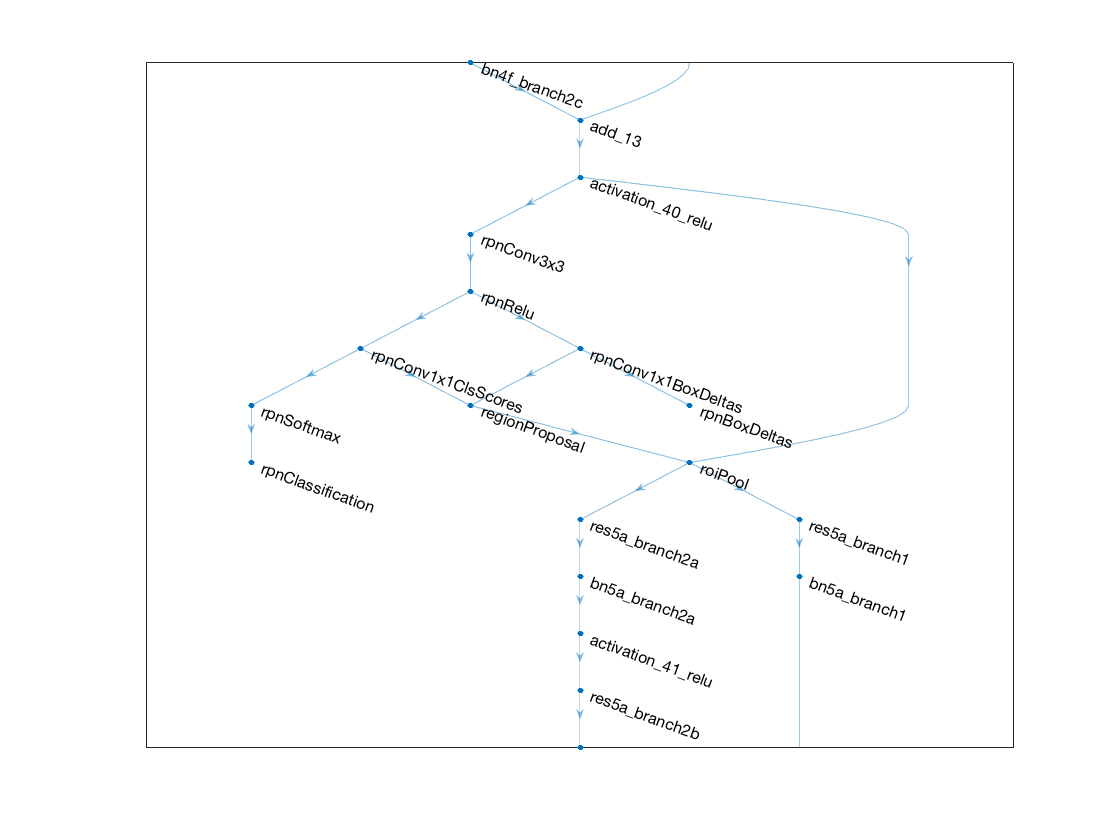The network is ready to be trained using `trainFasterRCNNObjectDetector`.